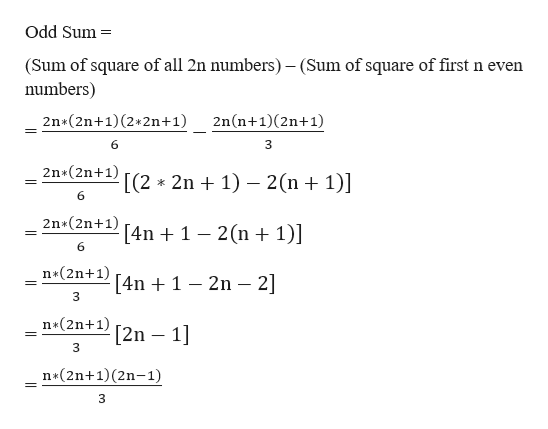# What is the units' digit of the sum of the squares of the first 2019 odd positive integers?

Question
60 views

What is the units' digit of the sum of the squares of the first 2019 odd positive integers?

check_circle

star
star
star
star
star
1 Rating
Step 1

To find the sum of squares of the first 2019 odd positive integers.help_outlineImage TranscriptioncloseOdd Sum (Sum of square of all 2n numbers) - (Sum of square of first n even numbers) 2n*(2n+1) (2 2n+1) 2n(n+1)(2n+1) 6 2n*(2n+1) [(2* 2n 1) 2(n 1)] 6 2n*(2n+1) [4n1 2(n 1)] 6 n*(2n+ 1) [4n1 2n-2] n*(2n+1) [2n 1] n*(2n+1) (2n-1) fullscreen
Step 2

Now, to find the number of odd numbers until 2019.

Step 3

To substitute the value of n as 1...

### Want to see the full answer?

See Solution

#### Want to see this answer and more?

Solutions are written by subject experts who are available 24/7. Questions are typically answered within 1 hour.*

See Solution
*Response times may vary by subject and question.
Tagged in

### Equations and In-equations